# 我国P2P网贷平台融资效率及其影响因素研究Financing Efficiency and Influencing Factors of China’s P2P Online Lending Platform

• 全文下载: PDF(768KB)    PP.102-110   DOI: 10.12677/SSEM.2019.83015
• 下载量: 238  浏览量: 1,369

Since the first online loan platform “Pai Pai Dai” was registered and established in Shanghai in 2007, the online loan industry has experienced 10 years of rapid development, and the number of platforms and transaction scale have been greatly improved. As of December 2018, China has nearly 2,000 online P2P lending platforms, with a monthly transaction volume of over 100 billion yuan. In this paper, based on the data of 199 platforms adopted from online loan home from Jan. 2018 to Jun. 2018, and combining with DEA-Tobit two-stage research method, the efficiency of online loan platform and its influencing factors were studied. The research in this paper not only enriches the research content of China’s P2P online lending and expands the application scope of DEA-Tobit two-stage research method, but also has certain reference significance for China’s individual investors, online lending platforms and corresponding regulatory authorities.

1. 引言

2. P2P网贷平台融资效率的实证分析

2.1. 效率理论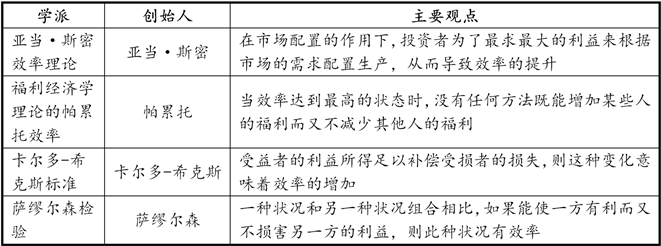Figure 1. Main ideas about efficiency

Farrell (1957)2采用最优生产前沿的方法对效率进行界定，并提出了X-效率；Chaenes (1978)和Fare (1985)3等人从投入角度和产出角度对效率进行了定义。有学者从X效率中划分技术效率(TE)，技术效率又可划分为纯技术效率(PTE)和规模效率(SE)。本文采取的效率研究方法(DEA)就是基于本项理论展开。考虑到我国P2P网贷平台正在处于规范业务、提升成交规模的快速发展阶段，BCC模型在CCR模型上去掉了规模报酬不变的假设限制，更贴合现实的生产经营场景，故本文采取了规模报酬可变的BCC模型。

2.2. 研究设计

$每万元借款平均满标时间=\frac{标的平均满标时间}{标的平均金额}=\frac{标的平均满标时间}{平台成交量/平台借款标数}$ (2.1)

$满标时间指数=\frac{1}{每元借款平均满标时间}$ (2.2)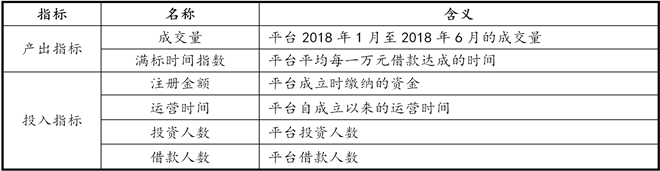Figure 2. Input and output indicators

2.3. 实证分析与结果讨论

1) 描述性统计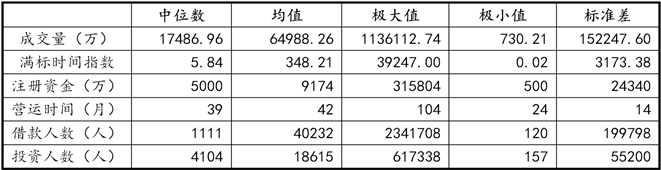Figure 3. Statistical test and analysis of input and output indicators

2) 实证结果与讨论

3. 融资效率影响因素的实证分析

3.1. Tobit模型理论

Tobit模型亦称为因变量受限模型，由诺贝尔经济学奖获得者James Tobin (1958)4提出，基本模型为：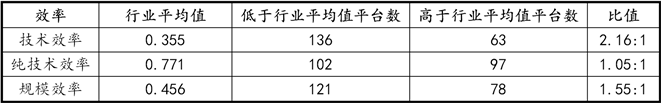Figure 4. Statistics of technical efficiency, pure technical efficiency and scale efficiency of 199 online loan platforms

${y}_{t}^{*}={\beta }^{\text{T}}{X}_{i}+{u}_{i},\text{\hspace{0.17em}}\text{\hspace{0.17em}}{u}_{i}~N\left(0,{\sigma }^{2}\right)$ (3.1)

${y}_{t}=\left\{\begin{array}{l}{y}_{t}^{*}\text{\hspace{0.17em}}\text{\hspace{0.17em}}\text{\hspace{0.17em}}\text{ }\text{ }\text{ }\text{if}\text{\hspace{0.17em}}{y}_{t}^{*}>0\\ 0\text{\hspace{0.17em}}\text{\hspace{0.17em}}\text{\hspace{0.17em}}\text{ }\text{\hspace{0.17em}}\text{\hspace{0.17em}}\text{if}\text{\hspace{0.17em}}{y}_{t}^{*}\le 0\end{array}$ (3.2)

${y}_{t}^{*}={\beta }^{\text{T}}{X}_{i}+{u}_{i},\text{\hspace{0.17em}}\text{\hspace{0.17em}}{u}_{i}~N\left(0,{\sigma }^{2}\right)$ (3.3)

${y}_{t}=\left\{\begin{array}{l}{c}_{t}^{+},\text{\hspace{0.17em}}\text{\hspace{0.17em}}\text{\hspace{0.17em}}\text{\hspace{0.17em}}\text{\hspace{0.17em}}\text{if}\text{\hspace{0.17em}}{y}_{t}^{*}>{c}_{t}^{+}\\ {y}_{t}^{*},\text{\hspace{0.17em}}\text{\hspace{0.17em}}\text{\hspace{0.17em}}\text{\hspace{0.17em}}\text{\hspace{0.17em}}\text{if}\text{\hspace{0.17em}}{c}_{t}^{-}\le {y}_{t}^{*}\le {c}_{t}^{+}\\ {c}_{t}^{-},\text{\hspace{0.17em}}\text{\hspace{0.17em}}\text{\hspace{0.17em}}\text{\hspace{0.17em}}\text{\hspace{0.17em}}\text{if}\text{\hspace{0.17em}}{y}_{t}^{*}<{c}_{t}^{-}\end{array}$ (3.4)

Tobit模型采用最大似然估计方法进行估计，其基本模型为：

$L=\underset{{y}_{i}}{\prod }\left(1-{F}_{i}\right)\underset{{y}_{i}>0}{\prod }\frac{1}{\sqrt{2\text{π}}}{\text{e}}^{-\frac{1}{2{\sigma }^{2}}{\left({y}_{t}-{\beta }^{\text{T}}x\right)}^{2}}$ (3.5)

${F}_{i}={\int }_{-\infty }^{{\beta }^{\text{T}}\frac{{x}_{i}}{\sigma }}\frac{1}{\sqrt{2\text{π}}}{\text{e}}^{-\frac{{t}^{2}}{2}}\text{d}t$ (3.6)

3.2. 研究设计

1) 样本及变量选取

2) 多重共线性检验

1、相关系数的检验

2、方差膨胀因子(VIF)检验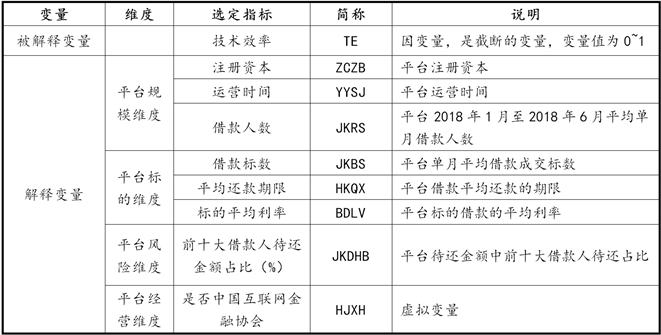Figure 5. Selection of explanatory variables and explained variables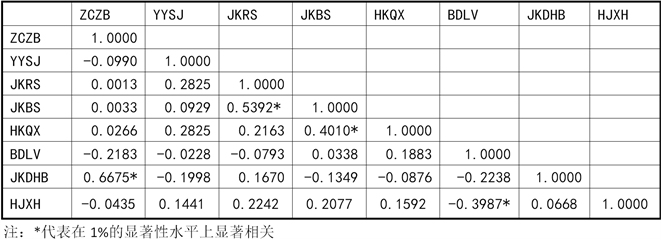Figure 6. Correlation coefficient test among variables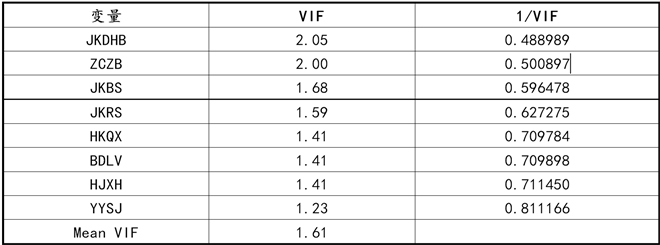Figure 7. Variance inflation factor (VIF) test

3) 研究假设

1、平台经营维度

H1：平台的注册资本与平台的融资效率具有正相关关系。

H2：平台的运营时间与平台的融资效率具有正相关关系。

H3：平台的月借款人数与平台的融资效率具有正相关关系。

2、平台标的维度

H4：平台借款标数与平台融资效率具有正相关关系。

H5：平台借款利率与平台融资效率成具有负相关关系。

H6：标的平均还款期限与平台融资效率具有负相关关系。

3、平台风险维度

H7：平台前十大借款人贷款待还占比与融资效率具有负相关关系。

4、平台经营维度

2016年3月互联网金融协会在上海正式揭牌成立，能够入选互联网金融协会是平台综合实力的反映，对平台的融资效率有一定影响，因此，本文提出假设H8：

H8：平台加入互联网金融协会能促进网贷平台融资效率的提升。

3.3. 实证分析及结果讨论

1) 回归模型设定

$\begin{array}{c}{\text{TE}}_{i}={\beta }_{0}+{\beta }_{1}{\text{ZCZB}}_{i}+{\beta }_{2}{\text{YYSJ}}_{i}+{\beta }_{3}{\text{JKRS}}_{i}+{\beta }_{4}{\text{JKBS}}_{i}+{\beta }_{5}{\text{HKQX}}_{i}\\ \text{\hspace{0.17em}}\text{\hspace{0.17em}}+{\beta }_{6}{\text{BDLV}}_{i}+{\beta }_{7}{\text{JKDHB}}_{i}+{\beta }_{8}{\text{HJXH}}_{i}+{\epsilon }_{i}\end{array}$ (3.7)

2) 回归结果

3) 结果讨论

① 平台规模维度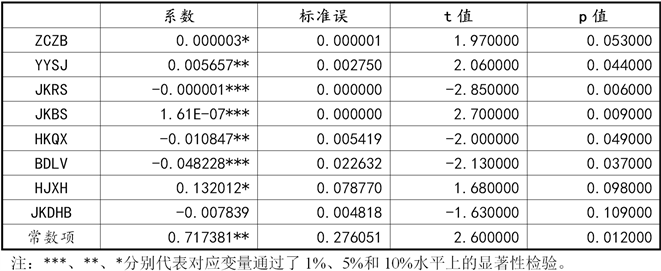Figure 8. Tobit regression result

② 平台标的维度：

③ 平台风险维度：

④ 平台经营维度：

4. 总结

NOTES

1图标说明：参考王文胜。中国商业银行经济效率研究。兰州大学出版社。2011年11月第一次印刷。第7页。

2Farrell, M.J. (1957) The Measurement of Productive Efficiency. Journal of the Royal Statistical Society, 120, 125-281.

3Fare, R., Grosskopf, S. and Knox, L.C.A. (1985) The Measurement of Efficiency of Production. Kluwer-Nijhoff, Boston.

4 Tobin, J. (1958) Estimation of Relationships for Limited Dependent Variable. The Econometric Society, 26, 24-36.

5转引自：汇付天下副总裁邹雯在2015中国互联网金融大会上的演讲：http://iof.hexun.com/2015-05-21/176029845.html (注：2015年汇付天下托管600多家P2P平台)。

  孙宝文, 欧阳日辉, 王天梅. 互联网金融元年：跨界、变革与融和[M]. 北京: 北京经济科学出版社, 2014.  周沛锋, 张宝明. 我国P2P小额信贷融资效率的研究[J]. 财会金融, 2014, 6(6): 51-53.  朱宗元, 王景裕. P2P网络借贷平台效率的综合评价——基于AHP-DEA方法[J]. 南方金融, 2016(4): 31-38.  邓思海, 田原, 金璐. 基于DEA方法的我国网贷平台运营效率研究[J]. 经济研究参考, 2016(20), 67-77.  王文胜. 商业银行经营效率研究[M]. 兰州: 兰州大学出版社, 2011: 6.  叶宗裕. 关于多项综合评价中指标正向化和无量纲化方法的选择[J]. 浙江统计, 2003(4): 24-25.